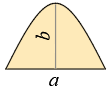### Area and Arc Length of Parabolic SegmentDimensions

Length - a =

Height - b =

Area

A = 23·a·b

Arc length

Assume h = ba

and q =  1 + h2

S = 0.5·a·(q + ln(h + q)h)